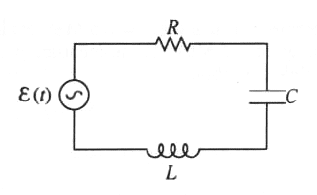GR 8677927796770177 | # Login | Register

GR9277 #81
Problem
 GREPhysics.NET Official Solution Alternate Solutions
\prob{81}In the RLC circuit shown above, the applied voltage is

.

For a constant , at what angular frequency does the current have its maximum steady-state amplitude after the transients have died out?

Electromagnetism}Resonant Frequency

The maximum steady-state amplitude (after transients die out) occurs at the resonant frequency, which is given by setting the impedance of the capacitor and inductor equal , as in choice (C).Alternate Solutions
 student20082008-10-16 10:47:38 We need to distinguish between the resonance of currents and that of other quantities (voltage, charge, etc.). The simplest way here is to write the equation for current in the complex form: , where , . Thus, . Since the sum of the squares is the smallest when the second square is equal to zero, we obtain (C). To the contrary, if we want, for example, to achieve the maximum voltage on the capacitor, or the maximum emf of the coil, we need to integrate or differentiate the expression for the current given above with respect to , and then take derivatives of modules of that expressions with respect ot . Thus, the frequency would be someway shifted, as in choice (D).Reply to this commentneon37
2010-11-12 06:29:46
Here we have a RLC driven circuit. And we are trying to find frequency, so isnt it the one that doesnt have R dependance?student2008
2008-10-16 10:47:38
We need to distinguish between the resonance of currents and that of other quantities (voltage, charge, etc.). The simplest way here is to write the equation for current in the complex form:
, where , .
Thus, . Since the sum of the squares is the smallest when the second square is equal to zero, we obtain (C).
To the contrary, if we want, for example, to achieve the maximum voltage on the capacitor, or the maximum emf of the coil, we need to integrate or differentiate the expression for the current given above with respect to , and then take derivatives of modules of that expressions with respect ot . Thus, the frequency would be someway shifted, as in choice (D).DaveyClaus
2006-11-28 19:36:09
I guess ETS likes solutions! Am I wrong or is D the actual solution for unspecified R, L, and C?
 freeform2006-11-28 20:49:26 Now I think I get this problem. Answer D is for underdamped circuit (R small) ONLY if the voltage source is a DC switched on (to charge capacitor) and then off--behavior is an exponentially decaying sinusoid. Here we have a continuously driving AC source, so the voltage should look just like a pure LC circuit (sinusoidal). Remember LRC circuits in electronics lab?
 pam d2011-09-30 17:27:26 This discussion is exactly why it is important they noted that the transients have died out. The general solution of a driven oscillator differential equation contains two parts, first is the solution to the homogeneous diffeq (in other words your LRC circuit without a driving voltage source) that is dependent upon initial conditions. This is the transient part because it dies off exponentially. The second part of the general solution is independent of initial conditions and is purely oscillatory. This is called the "steady state" solution because if you wait long enough (long enough for the transient part to decay away) you are going to end up with it alone. The steady state angular frequency for maximum current amplitude is (C) for reasons discussed by yosun and jax six years ago below.jax
2005-12-06 13:59:54
Isn't the impedance and , and then resonance occurs when so Z_C = -Z_L\omega\omega = \sqrt{\frac{1}{LC}}$I'm not sure if there is some case when you can do this the way it was done above without complex impedances, but this is the way I learned it.  jax2005-12-06 14:00:41 Isn't the impedance and , and then resonance occurs when so . Then if you solve for you get I'm not sure if there is some case when you can do this the way it was done above without complex impedances, but this is the way I learned it.  yosun2005-12-06 19:44:48 jax: actually, I believe impedance is given by the modulus of , and resonance occurs when for a minimal impedance. The and don't involve the imaginary term, per se.  jax2005-12-06 21:31:32 I guess that condition () holds for resonance, but you're setting the reactance (X) equal, not the impedance (Z). If you want the condition for resonance in terms of impedance (Z) see my previous post. I'm just getting all confused with reactance vs. impedance now! These websites are useful... http://en.wikipedia.org/wiki/Reactance /> http://en.wikipedia.org/wiki/Impedance /> http://en.wikipedia.org/wiki/RLC_circuit  yosun2005-12-06 23:42:16 right... the impedance isn't 0 since there will always be some resistance (although negligibly small sometimes). resonance isn't impedance matching, per se. see http://grephysics.net/disp.php?yload=prob&serial=1&prob=64 for another application of resonance.Post A Comment!  Username: Password: Click here to register. This comment is best classified as a: (mouseover)Mouseover the respective type above for an explanation of each type. ## Bare Basic LaTeX Rosetta Stone LaTeX syntax supported through dollar sign wrappers$, ex., $\alpha^2_0$ produces .
type this... to get...
$\int_0^\infty$
$\partial$
$\Rightarrow$
$\ddot{x},\dot{x}$
$\sqrt{z}$
$\langle my \rangle$
$\left( abacadabra \right)_{me}$
$\vec{E}$
$\frac{a}{b}$

The Sidebar Chatbox...
Scroll to see it, or resize your browser to ignore it...